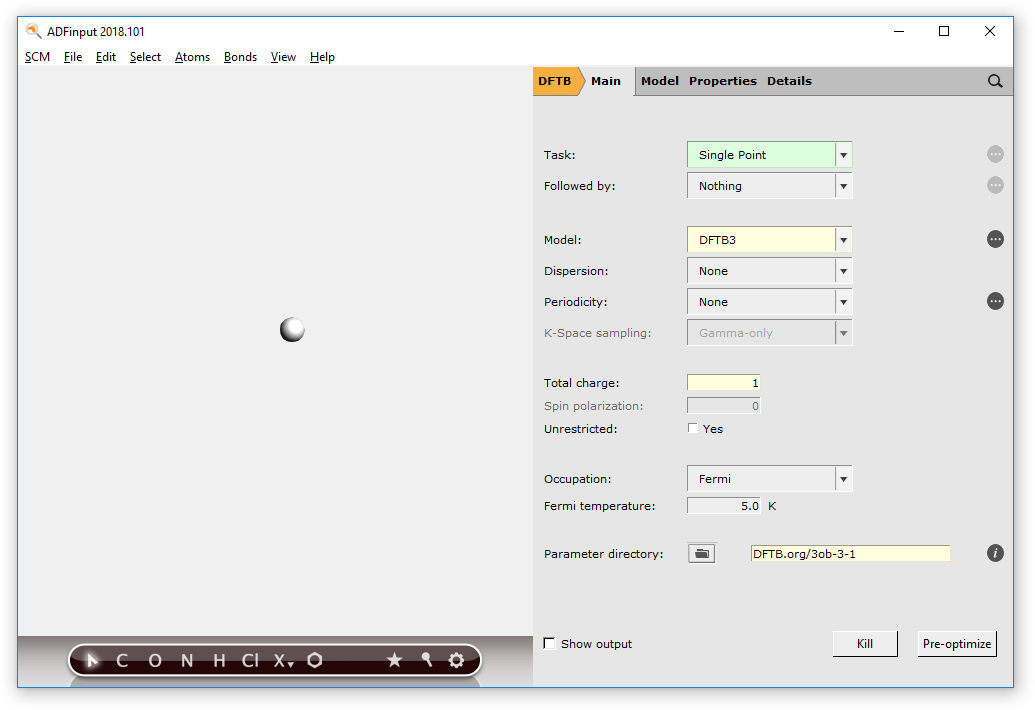# Proton affinities with DFTB3¶

In this tutorial we will calculate a proton affinity, defined as the negative gas phase enthalpy for the protonation reaction A- + H+ → AH. Gaus, Cui and Elstner have shown that third-order terms (DFTB3) generally improve the proton affinities with respect to second order self-consistent charges (SCC).

We will calculate the proton affinity of the acetate anion, CH3 COO- . In the first step of this tutorial, we will optimize acetic acid at the DFTB3 level. The second step will perform the computation on the anionic species, and compute its proton affinity.

## Step 1: Optimization of the neutral molecule¶

SCM → New Input.
Switch to DFTB mode (panel bar ADF → DFTB).

Now we need to obtain an acetic acid molecule and set up a geometry optimization at the DFTB3 level.

Use the Structure tool (hexagon in the bar below the molecule view), select Solvents → Acetic acid.
Click anywhere in the visualization pane. The acetic acid molecule will appear.
Make sure Geometry optimization is selected as the task to perform.
Select DFTB3 as the model.
Select the DFTB.org/3ob-3-1 parameter directory.
Save and run the job either through File → Run or by pressing ctrl+r. You will be asked to give your job a name.When the optimization is complete (it should not take longer than a second), ADFInput will ask if you want to update your geometry with the optimized geometry. For this tutorial it does not matter if you load the new geometry or not, as we are not going to run any calculations with the optimized geometry.

Open the output file through SCM → Output.
Scroll down through the steps of the geometry optimization until you find the message: Optimization Converged.
Below that you find a printout of the converged geometry, as well as energies, gradients, Mulliken charges and orbitals. Look for the line with Total energy (Hartree) and write down the value.

The total energy of the acetic acid molecule should be close to E(HAc) = -11.5755 Hartree. In order to calculate the proton affinity we now need to detach a proton and calculate the energies of the acetate ion and the lone proton.

## Step 2: Optimization of the acetate and the hydrogen ions¶

In order to perform the calculation on the acetate ion, we will remove the hydrogen ion from the previously computed acetic acid molecule.

Select the hydrogen in the COOH group by clicking on it.
Press the Backspace key on your keyboard. This will delete the hydrogen atom.
Select the Main panel.
Set the Total charge to -1.0
Use File → Save As... to save your new job under a different name. Use File → Run or ctrl+r to run it.
Look for the Total energy (Hartree) in the output like you did for the neutral molecule.You should obtain an energy of about E(Ac- ) = -11.2458 Hartree for the acetate ion.

Finally we also need the energy of a lone proton. There is no point in performing a geometry optimization for a single proton, so here we simply perform a single point calculation.

Select and delete every atom of the molecule, except one hydrogen atom.
Select the Main panel.
Set the task to Single Point.
Set the charge to 1.0
Use File → Save As... to save your new job under a different name. Use File → Run or ctrl+r to run it.
Look for the Total energy (Hartree) in the output.The total energy of the proton should be close to E(H+ ) = 0.2407 Hartree.

The proton affinity is computed as PA = E(Ac- ) + E(H+ ) - E(HAc), resulting in a final proton affinity of 0.5704 Hartree, or 357.93 kcal/mol.

We leave it as an example to calculate the PA with DFTB2 (SCC-DFTB), and compare it with the high-level ab initio results that are also quoted in the DFTB3 paper.